# 在算法交易中 Kohonen 神经网络的实际应用。 第 I 部分 工具

18 二月 2019, 07:46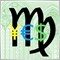0
470

### 修正错误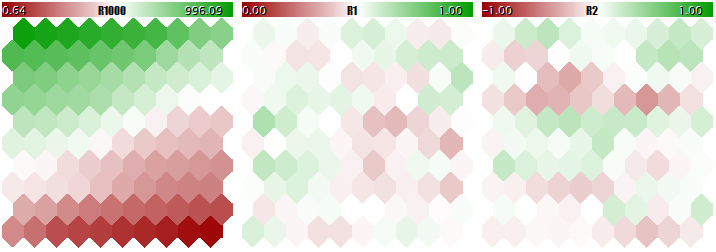Kohonen 网络学习结果未经输入常规化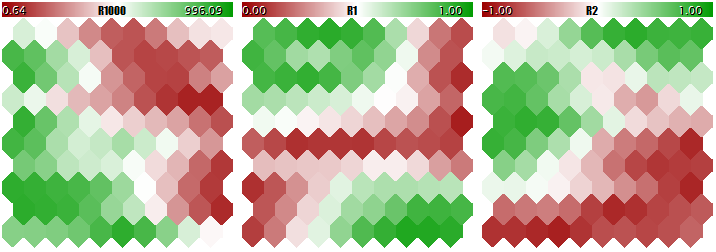Kohonen 网络输入常规化的学习结果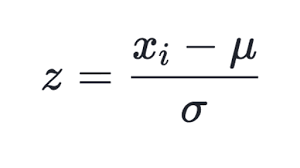(1)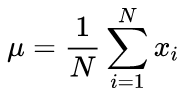(2)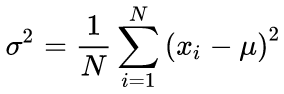(3)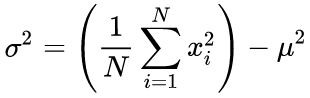(4)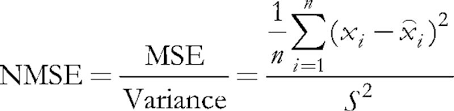(5)

N ~ 5 * sqrt(M)          (6)

S = sqrt(5 * sqrt(M))  (7)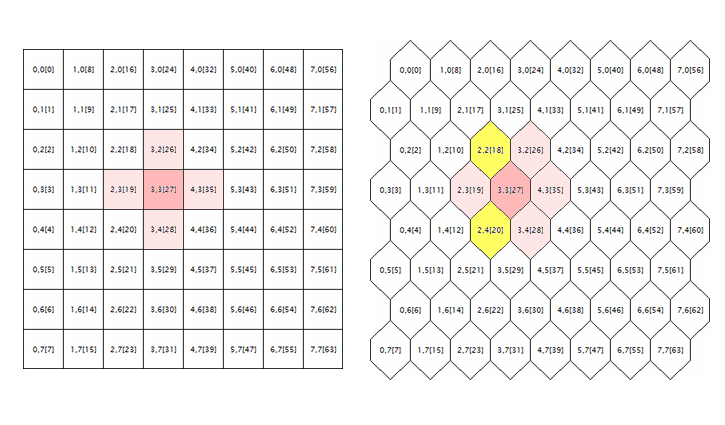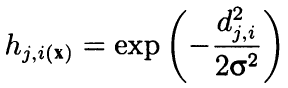(8)

```      for(int i = 0; i < total_nodes; i++)
{
double DistToNodeSqr = (m_som_nodes[winningnode].X() - m_som_nodes[i].X()) * (m_som_nodes[winningnode].X() - m_som_nodes[i].X())
+ (m_som_nodes[winningnode].Y() - m_som_nodes[i].Y()) * (m_som_nodes[winningnode].Y() - m_som_nodes[i].Y());
```

```      bool odd = ((winningnode % m_ycells) % 2) == 1;
for(int i = 0; i < total_nodes; i++)
{
bool odd_i = ((i % m_ycells) % 2) == 1;
double shiftx = 0;

if(m_hexCells && odd != odd_i)
{
if(odd && !odd_i)
{
shiftx = +0.5;
}
else // 反之亦然 (!odd && odd_i)
{
shiftx = -0.5;
}
}
double DistToNodeSqr = (m_node[winningnode].GetX() - (m_node[i].GetX() + shiftx)) * (m_node[winningnode].GetX() - (m_node[i].GetX() + shiftx))
+ (m_node[winningnode].GetY() - m_node[i].GetY()) * (m_node[winningnode].GetY() - m_node[i].GetY());
```

```        if(DistToNodeSqr < WS)
{
double influence = MathExp(-DistToNodeSqr / (2 * WS));
}
```

```        if(DistToNodeSqr < 9 * WS)
```

### 改进

```#define EXTRA_DIMENSIONS 5
#define DIM_HITCOUNT (m_dimension + 0)
#define DIM_UMATRIX  (m_dimension + 1)
#define DIM_NODEMSE  (m_dimension + 2) // 每个节点的量化误差：平均方差（标准差的平方）
#define DIM_CLUSTERS (m_dimension + 3)
#define DIM_OUTPUT   (m_dimension + 4)
```

U 型矩阵

```double CSOMNode::CalculateDistance(const CSOMNode *other) const
{
double vector[];
other.GetCodeVector(vector);
return CalculateDistance(vector);
}
```

```#define NBH_SQUARE_SIZE    4
#define NBH_HEXAGONAL_SIZE 6

template<typename T>
class Neighbourhood
{
protected:
int neighbours[];
int nbhsize;
bool hex;
int m_ycells;

public:
Neighbourhood(const bool _hex, const int ysize)
{
hex = _hex;
m_ycells = ysize;

if(hex)
{
nbhsize = NBH_HEXAGONAL_SIZE;
ArrayResize(neighbours, NBH_HEXAGONAL_SIZE);
neighbours = -1; // 上 (可视)
neighbours = +1; // 下 (可视)
neighbours = -m_ycells; // 左
neighbours = +m_ycells; // 右
/* 模板，在下面的循环中动态应用
// 奇数行
neighbours = -m_ycells - 1; // 左上
neighbours = -m_ycells + 1; // 左下
// 偶数行
neighbours = +m_ycells - 1; // 右上
neighbours = +m_ycells + 1; // 右下
*/
}
else
{
nbhsize = NBH_SQUARE_SIZE;
ArrayResize(neighbours, NBH_SQUARE_SIZE);
neighbours = -1; // 上 (可视)
neighbours = +1; // 下 (可视)
neighbours = -m_ycells; // 左
neighbours = +m_ycells; // 右
}

}
~Neighbourhood()
{
ArrayResize(neighbours, 0);
}

T loop(const int ind, const CSOMNode &p_node[])
{
int nodes = ArraySize(p_node);
int j = ind % m_ycells;

if(hex)
{
int oddy = ((j % 2) == 1) ? -1 : +1;
neighbours = oddy * m_ycells - 1;
neighbours = oddy * m_ycells + 1;
}

reset();

for(int k = 0; k < nbhsize; k++)
{
if(ind + neighbours[k] >= 0 && ind + neighbours[k] < nodes)
{
// 跳过包边
if(j == 0) // 顶行
{
if(k == 0 || k == 4) continue;
}
else if(j == m_ycells - 1) // 底行
{
if(k == 1 || k == 5) continue;
}

iterate(p_node[ind], p_node[ind + neighbours[k]]);
}
}

return getResult();
}

virtual void reset() = 0;
virtual void iterate(const CSOMNode &node1, const CSOMNode &node2) = 0;
virtual T getResult() const = 0;
};
```

```class UMatrixNeighbourhood: public Neighbourhood<double>
{
private:
int n;
double d;

public:
UMatrixNeighbourhood(const bool _hex, const int ysize): Neighbourhood(_hex, ysize)
{
}

virtual void reset() override
{
n = 0;
d = 0.0;
}

virtual void iterate(const CSOMNode &node1, const CSOMNode &node2) override
{
d += node1.CalculateDistance(&node2);
n++;
}

virtual double getResult() const override
{
return d / n;
}
};
```

```void CSOM::CalculateDistances()
{
UMatrixNeighbourhood umnh(m_hexCells, m_ycells);

for(int i = 0; i < m_xcells * m_ycells; i++)
{
double d = umnh.loop(i, m_node);

if(d > m_max[DIM_UMATRIX])
{
m_max[DIM_UMATRIX] = d;
}

m_node[i].SetDistance(d);
}
}
```

```void CSOMNode::RegisterPatternHit(const double &vector[])
{
m_hitCount++;
double e = 0;
for(int i = 0; i < m_dimension; i++)
{
m_sum[i] += vector[i];
m_sumP2[i] += vector[i] * vector[i];
e += (m_weights[i] - vector[i]) * (m_weights[i] - vector[i]);
}
m_mse += e / m_dimension;
}
```

```double CSOM::AddPatternStats(const double &data[])
{
static double vector[];
ArrayCopy(vector, data);

int ind = GetBestMatchingIndex(vector);

m_node[ind].RegisterPatternHit(vector);

double code[];
m_node[ind].GetCodeVector(code);
Denormalize(code);

double mse = 0;

for(int i = 0; i < m_dimension; i++)
{
mse += (data[i] - code[i]) * (data[i] - code[i]);
}

mse /= m_dimension;

return mse;
}
```

```double CSOM::CalculateStats(const bool complete = true)
{
double data[];
ArrayResize(data, m_dimension);
double trainedMSE = 0.0;

for(int i = complete ? 0 : m_validationOffset; i < m_nSet; i++)
{
ArrayCopy(data, m_set, 0, m_dimension * i, m_dimension);
trainedMSE += AddPatternStats(data, complete);
}

double nmse = trainedMSE / m_dataMSE;
if(complete) Print("Overall NMSE=", nmse);

return nmse;
}
```

```void CSOM::CalculateDataMSE()
{
double data[];

m_dataMSE = 0.0;

for(int i = m_validationOffset; i < m_nSet; i++)
{
ArrayCopy(data, m_set, 0, m_dimension * i, m_dimension);

double mse = 0;
for(int k = 0; k < m_dimension; k++)
{
mse += (data[k] - m_mean[k]) * (data[k] - m_mean[k]);
}

mse /= m_dimension;
m_dataMSE += mse;
}
}
```

```void CSOM::InitNormalization(const bool normalization = true)
{
ArrayResize(m_max, m_dimension + EXTRA_DIMENSIONS);
ArrayResize(m_min, m_dimension + EXTRA_DIMENSIONS);
ArrayInitialize(m_max, 0);
ArrayInitialize(m_min, 0);
ArrayResize(m_mean, m_dimension);
ArrayResize(m_sigma, m_dimension);

for(int j = 0; j < m_dimension; j++)
{
double maxv = -DBL_MAX;
double minv = +DBL_MAX;

if(normalization)
{
m_mean[j] = 0;
m_sigma[j] = 0;
}

for(int i = 0; i < m_nSet; i++)
{
double v = m_set[m_dimension * i + j];
if(v > maxv) maxv = v;
if(v < minv) minv = v;
if(normalization)
{
m_mean[j] += v;
m_sigma[j] += v * v;
}
}

m_max[j] = maxv;
m_min[j] = minv;

if(normalization && m_nSet > 0)
{
m_mean[j] /= m_nSet;
m_sigma[j] = MathSqrt(m_sigma[j] / m_nSet - m_mean[j] * m_mean[j]);
}
else
{
m_mean[j] = 0;
m_sigma[j] = 1;
}
}
}
```

```int CSOMNode::GetHitsCount() const
{
return m_hitCount;
}

double CSOMNode::GetHitsMean(const int plane) const
{
if(m_hitCount == 0) return 0;
return m_sum[plane] / m_hitCount;
}

double CSOMNode::GetHitsDeviation(const int plane) const
{
if(m_hitCount == 0) return 0;
double z = m_sumP2[plane] / m_hitCount - m_sum[plane] / m_hitCount * m_sum[plane] / m_hitCount;
if(z < 0) return 0;
return MathSqrt(z);
}

double CSOMNode::GetMSE() const
{
if(m_hitCount == 0) return 0;
return m_mse / m_hitCount;
}
```

```double CSOMNode::CalculateOutput(const double &vector[])
{
m_output = CalculateDistance(vector);
return m_output;
}
```

```void CSOM::CalculateOutput(const double &vector[], const bool normalize = false)
{
double temp[];
ArrayCopy(temp, vector);
if(normalize) Normalize(temp);
m_min[DIM_OUTPUT] = DBL_MAX;
m_max[DIM_OUTPUT] = -DBL_MAX;
for(int i = 0; i < ArraySize(m_node); i++)
{
double x = m_node[i].CalculateOutput(temp);
if(x < m_min[DIM_OUTPUT]) m_min[DIM_OUTPUT] = x;
if(x > m_max[DIM_OUTPUT]) m_max[DIM_OUTPUT] = x;
}
}
```

K 均值

`#include <Math/Alglib/dataanalysis.mqh>`

```void CSOM::Clusterize(const int clusterNumber)
{
int count = m_xcells * m_ycells;
CMatrixDouble xy(count, m_dimension);
int info;
CMatrixDouble clusters;
int membership[];
double weights[];

for(int i = 0; i < count; i++)
{
m_node[i].GetCodeVector(weights);
xy[i] = weights;
}

CKMeans::KMeansGenerate(xy, count, m_dimension, clusterNumber, KMEANS_RETRY_NUMBER, info, clusters, membership);
Print("KMeans result: ", info);
if(info == 1) // ok
{
for(int i = 0; i < m_xcells * m_ycells; i++)
{
m_node[i].SetCluster(membership[i]);
}

ArrayResize(m_clusters, clusterNumber * m_dimension);
for(int j = 0; j < clusterNumber; j++)
{
for(int i = 0; i < m_dimension; i++)
{
m_clusters[j * m_dimension + i] = clusters[i][j];
}
}
}
}
```

`m_node[i].SetCluster(membership[i]);`

• 如果不是，我们创建一个新的聚类，并将当前的神经元赋予它。 请注意，将创建聚类，从零索引开始，该零索引对应于最“重要”的聚类，因为它与最小 U 型距离匹配，然后按重要性降序排列。 就 U 型距离而言，每个连续的聚类将不那么紧凑。
• 如果在相邻神经元中那些有聚类标记的，我们将在它们中选择最高的一个，即 ，索引最低的那个，并将当前神经元分配给该聚类。

```double CSOMNode::GetMSE() const
{
if(m_hitCount == 0) return 0;
return m_mse / m_hitCount;
}
```

```class ClusterNeighbourhood: public Neighbourhood<int>
{
private:
int cluster;

public:
ClusterNeighbourhood(const bool _hex, const int ysize): Neighbourhood(_hex, ysize)
{
}

virtual void reset() override
{
cluster = -1;
}

virtual void iterate(const CSOMNode &node1, const CSOMNode &node2) override
{
int x = node2.GetCluster();
if(x > -1)
{
if(cluster != -1) cluster = MathMin(cluster, x);
else cluster = x;
}
}

virtual int getResult() const override
{
return cluster;
}
};
```

```void CSOM::Clusterize()
{
double array[];
int n = m_xcells * m_ycells;
ArrayResize(array, n);
for(int i = 0; i < n; i++)
{
if(m_node[i].GetHitsCount() > 0)
{
array[i] = m_node[i].GetDistance() * MathSqrt(m_node[i].GetMSE());
}
else
{
array[i] = DBL_MAX;
}
array[i] = i;
m_node[i].SetCluster(-1);
}
ArraySort(array);

ClusterNeighbourhood clnh(m_hexCells, m_ycells);

int count = 0; // 聚类数量
ArrayResize(m_clusters, 0);

for(int i = 0; i < n; i++)
{
// 如果已经分配则跳过
if(m_node[(int)array[i]].GetCluster() > -1) continue;

// 检查当前节点是否与任何现有聚类相邻
int r = clnh.loop((int)array[i], m_node);
if(r > -1) // 邻居已经属于聚类
{
m_node[(int)array[i]].SetCluster(r);
}
else // 我们需要新的聚类
{
ArrayResize(m_clusters, (count + 1) * m_dimension);

double vector[];
m_node[(int)array[i]].GetCodeVector(vector);
ArrayCopy(m_clusters, vector, count * m_dimension, 0, m_dimension);

m_node[(int)array[i]].SetCluster(count++);
}
}
}
```

### 测试

• DataFileName — 含有教育或测试数据的文本文件的名称; CSOM 类支持 csv 格式，但稍后我们会在 EA 本身中添加从文件读取集合，由于分析其他 EA 的优化设置是“危险的”; 文件包含的输入被指定，此名称也用于网络教育之后保存网络，但用另一个扩展名（见下文）; 您可以指示或省略 csv 扩展名; 此名称可能包含 MQL5/Files 中的文件夹;

• NetFileName — 含扩展名 som 的自有格式二进制文件的名称; CSOM 类能够在这些文件中保存和读取网络; 如果有人需要修改要存储的数据结构，则需修改文件开头写入的签名中的版本号; 如果 NetFileName 为空，则 EA 在学习模式下工作，而如果指定了网络，则在测试模式下，即 ，在准备好的网络中显示输入; 您可以指示或省略 som 扩展名; 并名称可能包含 MQL5/Files 中的文件夹;

• 如果 DataFileName 和 NetFileName 都为空，EA 将随机生成演示 3D 数据集，并据其进行教育;

• 如果 NetFileName 中的网络名称正确，您可以在 DataFileName 中指明不存在的文件名称，例如只是 '?' 字符，这会导致 EA 生成测试数据的随机样本，定义范围保存在网络文件中（请注意，此信息对于已教育网络是必要的，以便在操作模式下正确常规化未知数据，网络投喂输入来自另一个定义范围的数值当然不会引发出错，但结果将是不可靠的; 例如，如果回撤值为负数，或者为其提供的成交数为负值，则难以期望网络能够正常工作。

• CellsX — 网格的水平尺寸（神经元的数量），默认为 10;

• CellsY — 网格的垂直尺寸（神经元的数量），默认为 10;

• HexagonalCell — 使用六边形网格的特征，默认为“true”; 对于矩形网格，切换到“false”;

• UseNormalization — 启用/禁用输入常规化; 默认为 'true'，建议不要禁用它;

• EpochNumber — 学习时代的数量; 默认为 100;

• ValidationSetPercent — 验证选择的尺度，以输入总数的百分比表示; 它默认为 0，即 ，验证被禁用; 如果使用它，推荐值约为 10;

• ClusterNumber — 聚类数量; 它默认为 1，这意味着我们的自适应聚类; 0 值禁用聚类; 高于 0 的数值使用 K-均值方法启动聚类; 学习后立即进行聚类; 且聚类保存到网络文件;

• ImageW — 每个映像（平面）的水平尺寸（以像素为单位），默认为 500;

• ImageH — 每个映像（平面）的垂直尺寸（以像素为单位），默认为 500;

• MaxPictures — 一行的映像数量; 它默认为 0，意即在连续行中显示映像模式，使用滚动选项（允许大映像）; 如果 MaxPictures 大于 0，则整个平面集显示在若干行中，每个映像的 MaxPictures 均位于其内（便于以较小比例一起查看所有映像）;

• ShowBorders — 启用/禁用绘制神经元之间的边界; 默认为“false”;

• ShowTitles — 启用/禁用显示具有神经元特征的文本，默认为“true”;

• ColorScheme — 选择 4 种配色方案中的一种; 默认 Blue_Green_Red（最丰富多彩的一个）;

• ShowProgress — 在学习期间启用/禁用动态更新网络映像; 它每秒执行 1 次; 默认是“true”;

• RandomSeed — 用于初始化随机数发生器的是一个整数; 默认为 0;
• SaveImages — 完成后保存网络映像的选项; 它也可以在学习之后和第一次启动之后使用; 默认为 “false”;

CSOMDisplay 类对象将在 EA 中执行所有操作。

`CSOMDisplay KohonenMap;`

```void OnInit()
{
ChartSetInteger(0, CHART_EVENT_MOUSE_MOVE, true);
EventSetMillisecondTimer(1);
}

void OnChartEvent(const int id, const long &lparam, const double &dparam, const string &sparam)
{
KohonenMap.OnChartEvent(id, lparam, dparam, sparam);
}
```

```void OnTimer()
{
EventKillTimer();

MathSrand(RandomSeed);

bool hasOneTestPattern = false;

if(NetFileName != "")
{
KohonenMap.DisplayInit(ImageW, ImageH, MaxPictures, ColorScheme, ShowBorders, ShowTitles);

Comment("Map ", NetFileName, " is loaded; size: ", KohonenMap.GetWidth(), "*", KohonenMap.GetHeight(), "; features: ", KohonenMap.GetFeatureCount());
```

```    if(DataFileName != "")
{
{

// 生成随机测试矢量
int n = KohonenMap.GetFeatureCount();
double min, max;
double v[];
ArrayResize(v, n);
for(int i = 0; i < n; i++)
{
KohonenMap.GetFeatureBounds(i, min, max);
v[i] = (max - min) * rand() / 32767 + min;
}
Print("Random Input:");
ArrayPrint(v);
double y[];
CSOMNode *node = KohonenMap.GetBestMatchingFeatures(v, y);
Print("Matched Node Output (", node.GetX(), ",", node.GetY(), "); Hits:", node.GetHitsCount(), "; Error:", node.GetMSE(),"; Cluster N", node.GetCluster(), ":");
ArrayPrint(y);
KohonenMap.CalculateOutput(v, true);
hasOneTestPattern = true;
}
}
```

```  }
else // 未提供网络文件，因此假定进行训练
{
if(DataFileName == "")
{
// 生成无缩放值的 3D 演示矢量 {[0,+1000], [0,+1], [-1,+1]}
// 将它们投喂到网络中以便比较有无常规化的结果
// 注意 标题应该是有效的 BMP 文件名
string titles[] = {"R1000", "R1", "R2"};
KohonenMap.AssignFeatureTitles(titles);
double x;
for(int i = 0; i < 1000; i++)
{
x = 1000.0 * rand() / 32767;
x = 1.0 * rand() / 32767;
x = -2.0 * rand() / 32767 + 1.0;
KohonenMap.AddPattern(x, StringFormat("%f %f %f", x, x, x));
}
}
```

```    else // 提供了一个数据文件
{
{
return;
}
}
```

```    KohonenMap.Init(CellsX, CellsY, ImageW, ImageH, MaxPictures, ColorScheme, HexagonalCell, ShowBorders, ShowTitles);

if(ValidationSetPercent > 0 && ValidationSetPercent < 50)
{
KohonenMap.SetValidationSection((int)(KohonenMap.GetDataCount() * (1.0 - ValidationSetPercent / 100.0)));
}

KohonenMap.Train(EpochNumber, UseNormalization, ShowProgress);

if(ClusterNumber > 1)
{
KohonenMap.Clusterize(ClusterNumber);
}
else
{
KohonenMap.Clusterize();
}
}
```

```  if(!hasOneTestPattern)
{
double vector[];
ArrayResize(vector, KohonenMap.GetFeatureCount());
ArrayInitialize(vector, 0);
KohonenMap.CalculateOutput(vector);
}
```

```  KohonenMap.Render(); // 将映像绘制到内部 BMP 缓冲区中
```

```  if(hasOneTestPattern)
KohonenMap.ShowAllPatterns();
else
KohonenMap.ShowAllNodes(); // 在 BMP 缓冲区中绘制细胞内标签
```

```  if(ClusterNumber != 0)
{
KohonenMap.ShowClusters(); // 标记聚类
}
```

```  KohonenMap.ShowBMP(SaveImages); // 在图表上将文件显示为位图图像，可选择保存到文件中
```

```  if(NetFileName == "")
{
KohonenMap.Save(KohonenMap.GetID());
}
}
```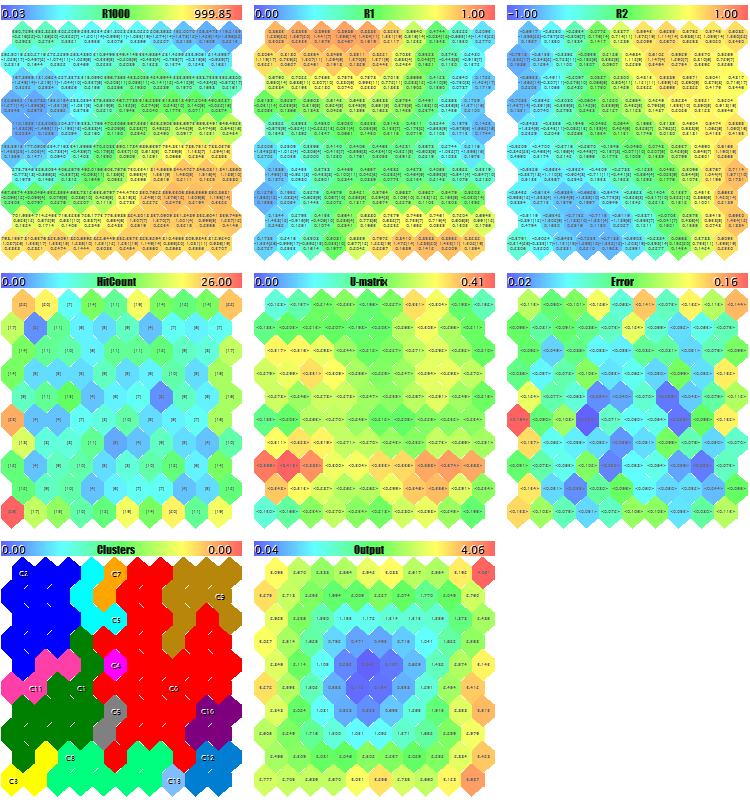```Pass 0 from 1000 0%
Pass 78 from 1000 7%
Pass 157 from 1000 15%
Pass 232 from 1000 23%
Pass 310 from 1000 31%
Pass 389 from 1000 38%
Pass 468 from 1000 46%
Pass 550 from 1000 55%
Pass 631 from 1000 63%
Pass 710 from 1000 71%
Pass 790 from 1000 79%
Pass 870 from 1000 87%
Pass 951 from 1000 95%
Overall NMSE=0.09420336270396877
Training completed at pass 1000, NMSE=0.09420336270396877
Clusters :
"R1000" "R1"    "R2"
N0
754.83131   0.36778   0.25369
N1
341.39665   0.41402  -0.26702
N2
360.72925   0.86826  -0.69173
N3
798.15569   0.17846  -0.37911
N4
470.30648   0.52326   0.06442
Map file SOM-20181205-134437.som saved
```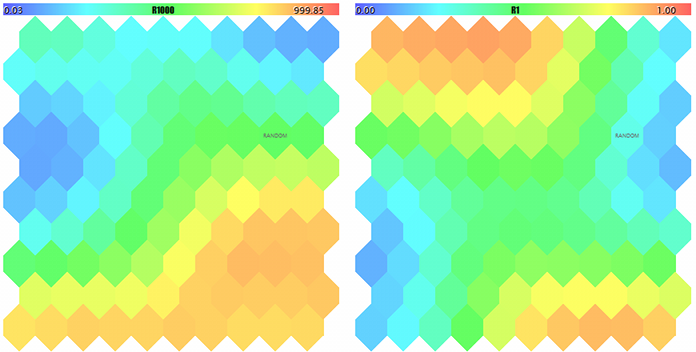Kohonen 映像随机 3D 矢量的前两个分量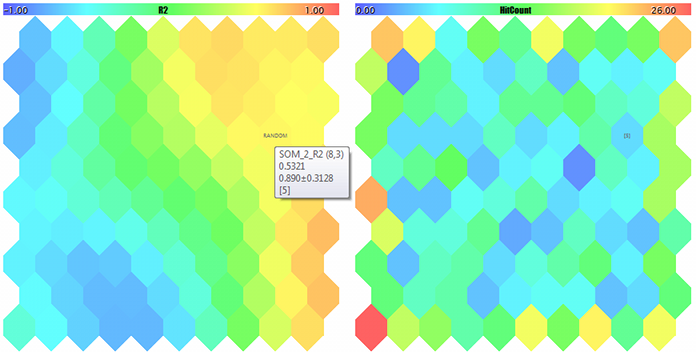Kohonen 映像，用于随机 3D 矢量的第三个分量和命中计数器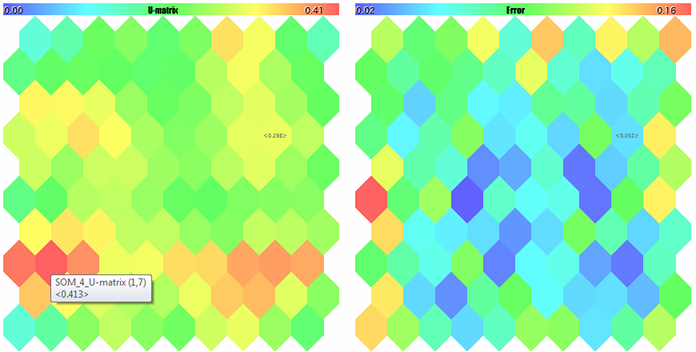U 型矩阵和量化误差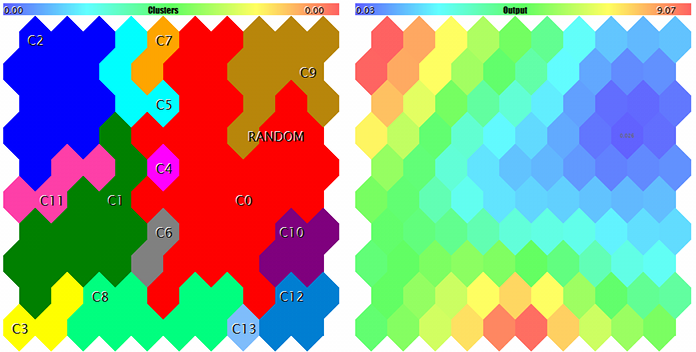```FileOpen error ?.csv : 5004
Random Input:
457.17510   0.29727   0.57621
Matched Node Output (8,3); Hits:5; Error:0.05246704285146882; Cluster N0:
497.20453   0.28675   0.53213
```

### 结束语

Kohonen1MQL5.zip (21.05 KB)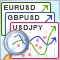利用 MQL5 和 MQL4 实现的选择和导航实用程序：添加"homework"选项卡并保存图形对象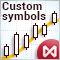如何在 MetaTrader 5 中创建并测试自定义 MOEX(莫斯科证券交易所) 品种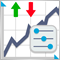分离策略在趋势和盘整条件下的优化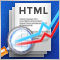利用 HTML 报告分析交易结果

MetaTrader 5 平台具有保存交易报告，以及智能交易系统测试和优化报告的功能。 交易和测试报告可以按照两种格式保存：XLSX 和 HTML，而优化报告可以保存为 XML。 在本文中，我们将研究 HTML 测试报告，XML 优化报告和 HTML 交易历史报告。